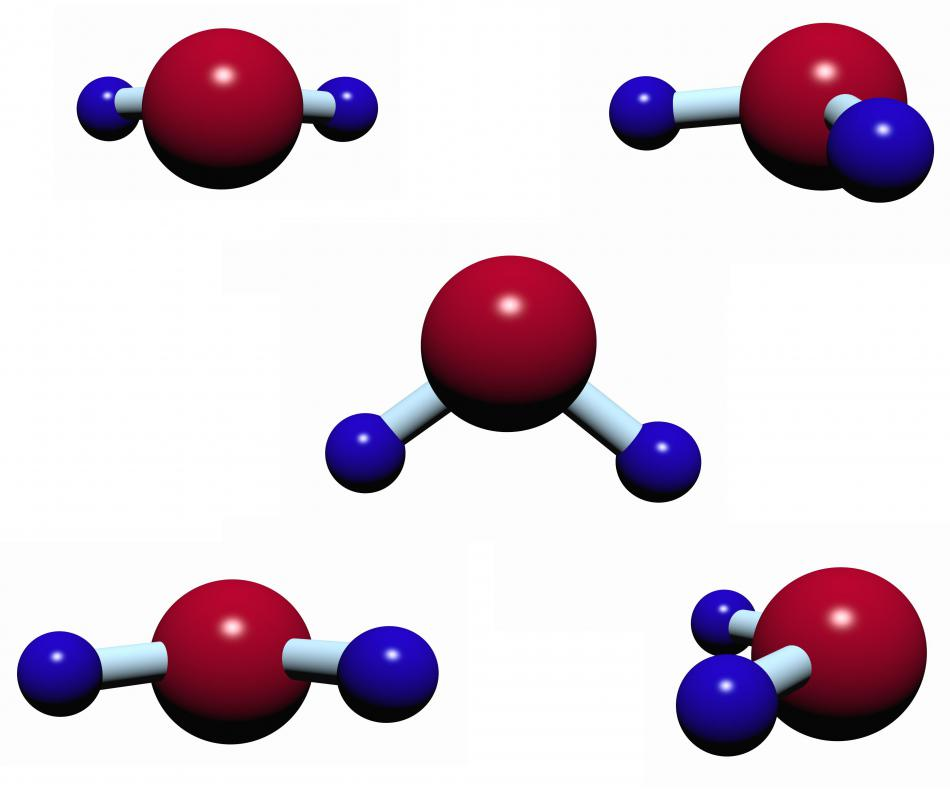What Is a Dipole Moment?

David Isaac Rudel
David Isaac RudelA dipole moment causes water molecules to spin in a microwave.

A dipole is a neutral system comprising two oppositely-charged parts. For example, a water molecule is neutral as a whole, but one of its ends is positively-charged while the other is negatively-charged. Such an object can influence other charged objects through electromagnetic forces. The dipole moment of a dipole is a vector quantity describing the strength of this influence. Its size equals the magnitude of each charge, multiplied by the distance between the systems two parts.

The strength of the force exerted by a dipole on a distant particle can be approximated using the equation F=2*pkq/r3. Here, p is the dipole moment, k is Coulomb’s constant, q is size of the net charge on the distant particle, and r is the separation between the center of the dipole and the distant particle. This approximation is nearly perfect on the longitudinal axis of the system so long as r is significantly larger than the separation between the two components of the dipole. For particles far away from this axis, the approximation overestimates the force by as much as a factor of 2.

Einstein’s theory of relativity links electric forces to magnetic forces. The magnetic field of a bar magnet can be approximated by a dipole of magnetic charges, one near the north pole of the magnet, the other near the south pole. Such an assemblage is called a magnetic dipole and the influence it exerts on a distant charge moving perpendicular to the field can be approximated as 2*μqs/r3, where μ is the magnetic dipole moment and s is the speed.

An electric current moving in a circular wire generates a magnetic field similar to that of a short bar magnet. The magnetic dipole moment of such a wire has magnitude I*A, where I is the wire’s current and A is the area it traces out in space. At the atomic level, magnetism is often viewed as arising from the motion of electrons along curved paths. The size of the magnetic dipole moment for such a particle equals q*s/(2r), where q is the size of the charge, s is the speed of the particle, and r is the radius of the path.

In addition to quantifying the force of a dipole on distant charged particles, the dipole moment is useful for determining the force an external field exerts on a dipole. For example, a microwave oven creates short-lived, varying electric fields. These fields cause water molecules, which are electric dipoles, to spin. This rotational motion leads to rising temperature, which cooks the food. The maximum torque exerted on a dipole by an external field is simply the product of the dipole moment and the field strength.

You might also Like